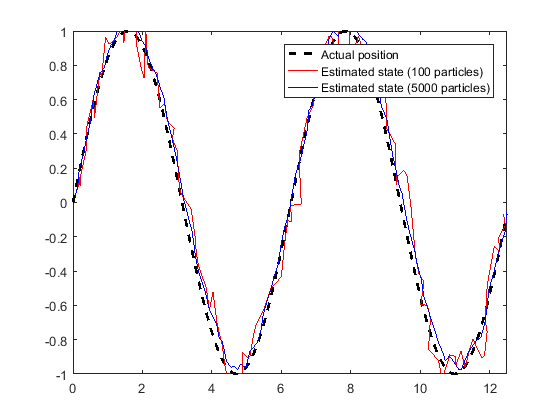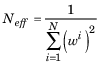## Particle Filter Parameters

To use the `stateEstimatorPF` (Robotics System Toolbox) particle filter, you must specify parameters such as the number of particles, the initial particle location, and the state estimation method. Also, if you have a specific motion and sensor model, you specify these parameters in the state transition function and measurement likelihood function, respectively. The details of these parameters are detailed on this page. For more information on the particle filter workflow, see Particle Filter Workflow.

### Number of Particles

To specify the number of particles, use the `initialize` method. Each particle is a hypothesis of the current state. The particles are distributed across your state space based on either a specified mean and covariance, or on the specified state bounds. Depending on the `StateEstimationMethod` property, either the particle with the highest weight or the mean of all particles is taken to determine the best state estimate.

The default number of particles is 1000. Unless performance is an issue, do not use fewer than 1000 particles. A higher number of particles can improve the estimate but sacrifices performance speed, because the algorithm has to process more particles. Tuning the number of particles is the best way to affect your particle filters performance.

These results, which are based on the `stateEstimatorPF` example, show the difference in tracking accuracy when using 100 particles and 5000 particles.### Initial Particle Location

When you initialize your particle filter, you can specify the initial location of the particles using:

• Mean and covariance

• State bounds

Your initial state is defined as a mean with a covariance relative to your system. This mean and covariance correlate to the initial location and uncertainty of your system. The `stateEstimatorPF` object distributes particles based on your covariance around the given mean. The algorithm uses this distribution of particles to get the best estimation of state, so an accurate initialization of particles helps to converge to the best state estimation quickly.

If an initial state is unknown, you can evenly distribute your particles across a given state bounds. The state bounds are the limits of your state. For example, when estimating the position of a robot, the state bounds are limited to the environment that the robot can actually inhabit. In general, an even distribution of particles is a less efficient way to initialize particles to improve the speed of convergence.

The plot shows how the mean and covariance specification can cluster particles much more effectively in a space rather than specifying the full state bounds.### State Transition Function

The state transition function, `StateTransitionFcn`, of a particle filter helps to evolve the particles to the next state. It is used during the prediction step of the Particle Filter Workflow. In the `stateEstimatorPF` object, the state transition function is specified as a callback function that takes the previous particles, and any other necessary parameters, and outputs the predicted location. The function header syntax is:

`function predictParticles = stateTransitionFcn(pf,prevParticles,varargin)`

By default, the state transition function assumes a Gaussian motion model with constant velocities. The function uses a Gaussian distribution to determine the position of the particles in the next time step.

For your application, it is important to have a state transition function that accurately describes how you expect the system to behave. To accurately evolve all the particles, you must develop and implement a motion model for your system. If particles are not distributed around the next state, the `stateEstimatorPF` object does not find an accurate estimate. Therefore, it is important to understand how your system can behave so that you can track it accurately.

You also must specify system noise in `StateTransitionFcn`. Without random noise applied to the predicted system, the particle filter does not function as intended.

Although you can predict many systems based on their previous state, sometimes the system can include extra information. The use of `varargin` in the function enables you to input any extra parameters that are relevant for predicting the next state. When you call `predict`, you can include these parameters using:

`predict(pf,param1,param2)`

Because these parameters match the state transition function you defined, calling `predict` essentially calls the function as:

`predictParticles = stateTransitionFcn(pf,prevParticles,param1,param2)`

The output particles, `predictParticles`, are then either used by the Measurement Likelihood Function to correct the particles, or used in the next prediction step if correction is not required.

### Measurement Likelihood Function

After predicting the next state, you can use measurements from sensors to correct your predicted state. By specifying a `MeasurementLikelihoodFcn` in the `stateEstimatorPF` object, you can correct your predicted particles using the `correct` function. This measurement likelihood function, by definition, gives a weight for the state hypotheses (your particles) based on a given measurement. Essentially, it gives you the likelihood that the observed measurement actually matches what each particle observes. This likelihood is used as a weight on the predicted particles to help with correcting them and getting the best estimation. Although the prediction step can prove accurate for a small number of intermediate steps, to get accurate tracking, use sensor observations to correct the particles frequently.

The specification of the `MeasurementLikelihoodFcn` is similar to the `StateTransitionFcn`. It is specified as a function handle in the properties of the `stateEstimatorPF` object. The function header syntax is:

`function likelihood = measurementLikelihoodFcn(pf,predictParticles,measurement,varargin)`

The output is the likelihood of each predicted particle based on the measurement given. However, you can also specify more parameters in `varargin`. The use of `varargin` in the function enables you to input any extra parameters that are relevant for correcting the predicted state. When you call `correct`, you can include these parameters using:

`correct(pf,measurement,param1,param2)`

These parameters match the measurement likelihood function you defined:

`likelihood = measurementLikelihoodFcn(pf,predictParticles,measurement,param1,param2)`

The `correct` function uses the `likelihood` output for particle resampling and giving the final state estimate.

### Resampling Policy

The resampling of particles is a vital step for continuous tracking of objects. It enables you to select particles based on the current state, instead of using the particle distribution given at initialization. By continuously resampling the particles around the current estimate, you can get more accurate tracking and improve long-term performance.

When you call `correct`, the particles used for state estimation can be resampled depending on the `ResamplingPolicy` property specified in the `stateEstimatorPF` object. This property is specified as a `resamplingPolicyPF` (Robotics System Toolbox) object. The `TriggerMethod` property on that object tells the particle filter which method to use for resampling.

You can trigger resampling at either a fixed interval or when a minimum effective particle ratio is reached. The fixed interval method resamples at a set number of iterations, which is specified in the `SamplingInterval` property. The minimum effective particle ratio is a measure of how well the current set of particles approximates the posterior distribution. The number of effective particles is calculated by:In this equation, N is the number of particles, and w is the normalized weight of each particle. The effective particle ratio is then Neff / `NumParticles`. Therefore, the effective particle ratio is a function of the weights of all the particles. After the weights of the particles reach a low enough value, they are not contributing to the state estimation. This low value triggers resampling, so the particles are closer to the current state estimation and have higher weights.

### State Estimation Method

The final step of the particle filter workflow is the selection of a single state estimate. The particles and their weights sampled across the distribution are used to give the best estimation of the actual state. However, you can use the particles information to get a single state estimate in multiple ways. With the `stateEstimatorPF` object, you can either choose the best estimate based on the particle with the highest weight or take a mean of all the particles. Specify the estimation method in the `StateEstimationMethod` property as either `'mean'`(default) or `'maxweight'`.

Because you can estimate the state from all of the particles in many ways, you can also extract each particle and its weight from the `stateEstimatorPF` using the `Particles` property.